9TH MAHARASHTRA: MATHS I 10TH TAMIL NADU: MATHS, SCIENCE, SSLC 10TH NEW TEXT BOOK PDF DOWNLOAD CBSE 10TH: MATHS 2019 BOARD PAPER SOLUTION: 2019 BOARD PAPER SOLUTION

### UNIT EXERCISE 1, RELATIONS AND FUNCTIONS, 10TH MATHS NEW SYLLABUS, TAMIL NADU, TN,

Question 1 :
If the ordered pairs (x2 − 3x, y2 + 4y) and (-2,5) are equal, then find x and y.
Solution :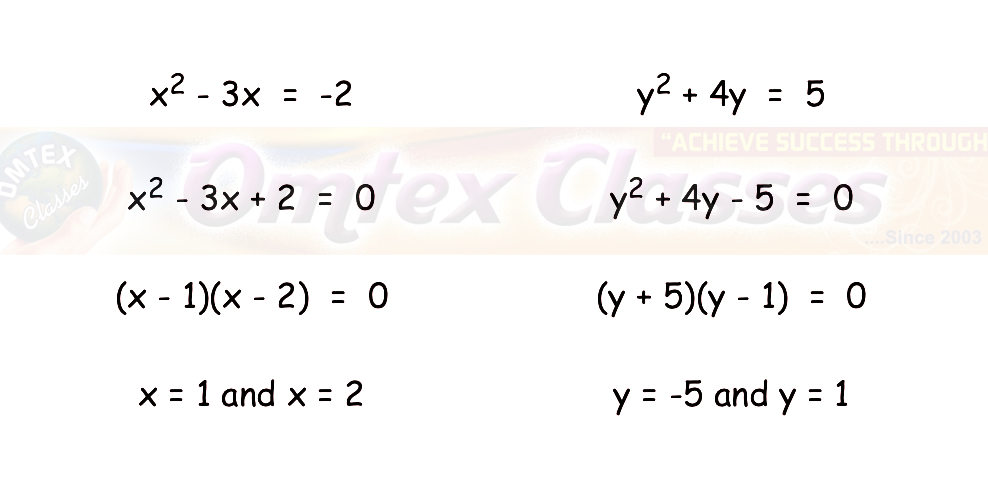Question 2 :
The Cartesian product A×A has 9 elements among which (–1, 0) and (0,1) are found. Find the set A and the remaining elements of A×A.

Solution :
So, in this question since A X A has 9 elements, so the number of elements in the set  A must obviously be 3.
Since the given elements of the cross product have -1, 0 and 1 as part of the entries, clearly these only must be the elements of the set A.
So A = {-1, 0, 1}
A X A
=  {(-1, -1),(-1, 0),(-1, 1),(0, -1),(0, 0),(0, 1),(1, -1),(1, 0),(1, 1)}

Question 3 :
Given that f(x)  =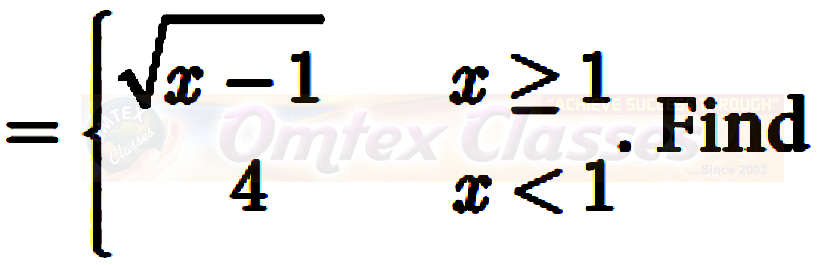(i) f (0) (ii) f (3) (iii) f (a+1) in terms of a.(Given that a   0)
Solution :
(i)  f(0)
Instead of x, we have 0, it is less than 1
f(0)  = 4
(ii)  f(3)
Instead of x, we have 3, it is greater than 1
f(3)  = √(3 - 1)
f(3)  = √2
(iii) f (a+1)
Instead of x, we have a+1, it is greater than 1
f(a + 1)  = √(a + 1 - 1)
f(a + 1)  = √a
Question 4 :
Let A= {9, 10, 11, 12, 13, 14, 15, 16, 17} and let f : A-> N be defined by f (n) = the highest prime factor of n A. Write f as a set of ordered pairs and find the range of f.
Solution :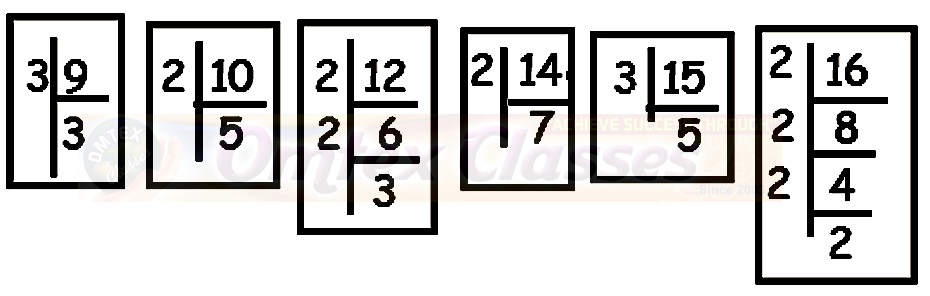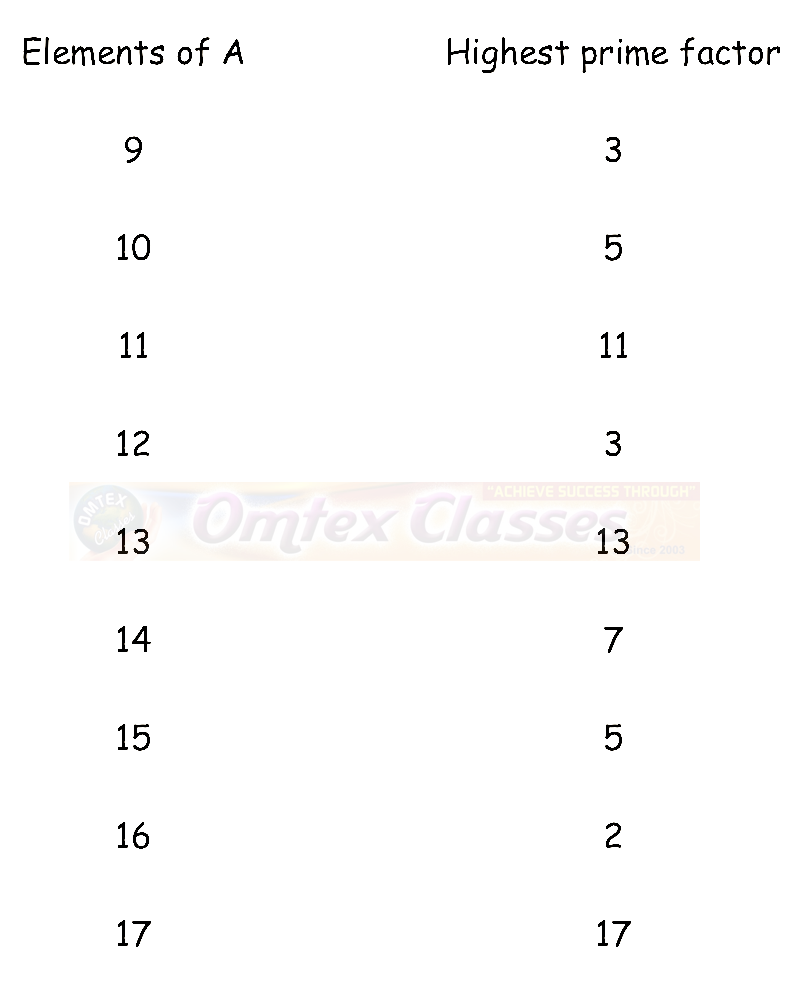Set ordered pairs :
=  {(9,3) (10, 5)(11, 11)(12, 3)(13, 13) (14, 7) (15, 5) (16,2) (17, 17)}
Range  = {2, 3, 5, 7,  11, 13, 17}

Question 5 :
Find the domain of the function f(x) =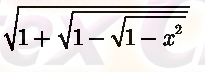Solution :
Let t = √(1 - √(1 - x2))
f(x)  = √(1 - t)
1 - t ≥ 0
t ≤ 1
By applying the value of t, we get
√(1 - √(1 - x2)) ≤ 1
1 - √(1 - x2)) ≤ 1
Subtracting 1 through out the equation,
- √(1 - x2)) ≤ 0
√(1 - x2)) ≥0
Taking squares on both sides,
(1 - x2)  ≥ 0
- x2 ≥ -1
x ≤ 1
Hence the domain is x ∈ [-1, 1]

Question 6 :
If f (x) = x2 , g(x) = 3x and h(x) = x −2 , Prove that (f g) h = f (g h) .
Solution :
L.H.S :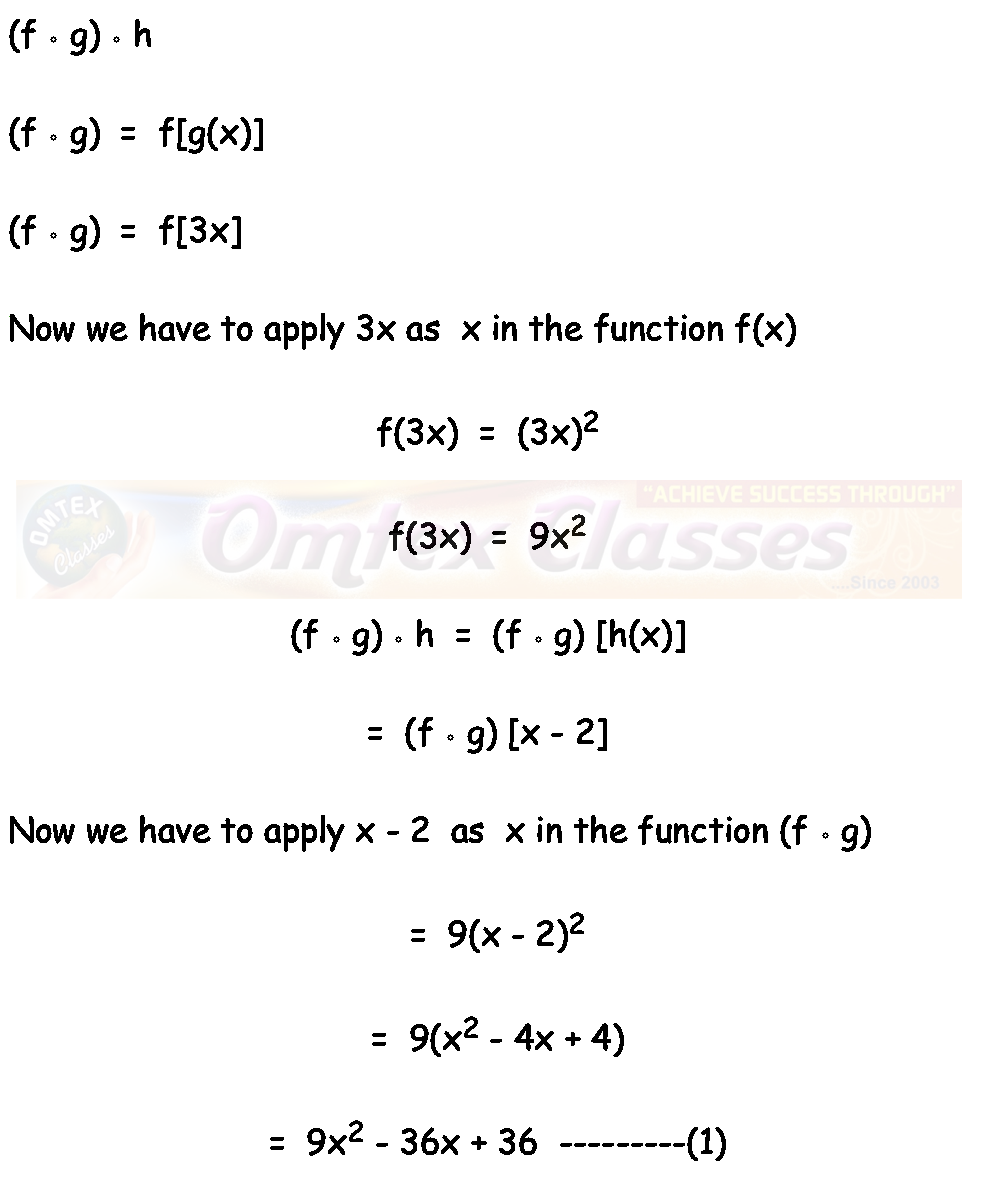R.H.S :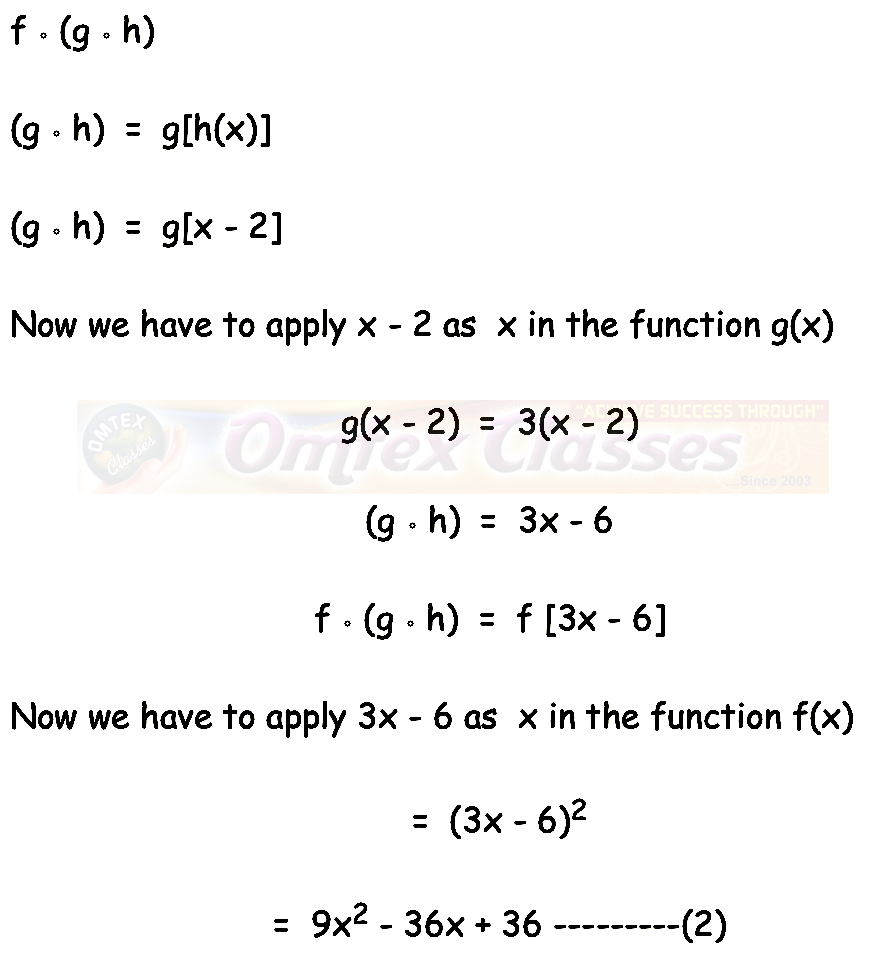Hence proved.

Question 7 :
Let A = {1, 2} and B = {1, 2, 3, 4} , C = {5, 6} and D = {5, 6 ,7, 8} . Verify whether A × C is a subset of B × D?
Solution :
A = {1, 2} and C = {5, 6}
A x C  = {(1, 5) (1, 6) (2, 5) (2, 6)}  ----(1)
B = {1, 2, 3, 4} and D = {5, 6 ,7, 8}
B x D  = { (1, 5) (1, 6) (1, 7) (1, 8) (2, 5) (2, 6) (2, 7) (2, 8) (3, 5) (3, 6) (3, 7) (3, 8) (4, 5) (4, 6) (4, 7) (4, 8) }
Hence A x C is the subset of B x D.

Question 8 :
If f(x)  = (x - 1)/(x + 1), x ≠ 1 show that f(f(x))  = -1/x, provided x ≠ 0
Solution :
f(x)  = (x - 1)/(x + 1) (Given)
f(f(x))  =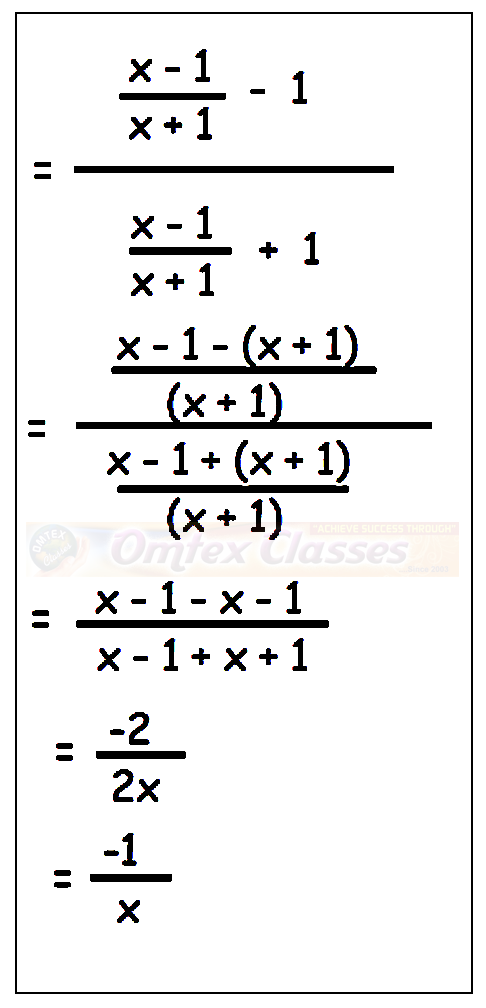Hence proved.

Question 9 :
The functions f and g are defined by f (x) = 6x + 8; g (x)  = (x - 2)/3
(i) Calculate the value of gg (1/2)
(ii) Write an expression for gf (x) in its simplest form.
Solution :
g (x)  = (x - 2)/3
g(1/2)  = ((1/2) - 2)/3
=  (-3/2)/3
g(1/2)   = -1/2
gg(1/2)  = ((-1/2) - 2)/3
=  (-5/2)/3
gg(1/2)  = -5/6

(ii) Write an expression for gf (x) in its simplest form.
gf (x)  = g[6x +8]
Now we have to apply 6x + 8 instead of x in g(x).
=  [(6x + 8) - 2]/3
=  (6x + 6)/3
=  2x + 2
=  2 (x + 1)

Question 10 :
Write the domain of the following real functions
(i) f (x)  = (2x + 1)/(x - 9)
Solution :
To find the domain, let us equate the denominator to 0
x - 9 = 0
x  = 9
The function is defined for all real values of x except 9.
Hence the required domain is R - {9}.

(ii)  p(x) =  -5/(4x2 + 1)
Solution :
In the denominator, we have x2. For all real values of x, we get positive values. Hence the required domain is R.

(iii)  g(x) =  √(x - 2)
Solution :
Since the given function is in radical sign, we should not get negative answer. For that we have to apply the values grater than 2.
Hence the required domain for he given function is [2, ∞)

(iv) h(x)  = x + 6
Solution :
For all real values of x, we get defined values of h(x). Hence the domain is R.